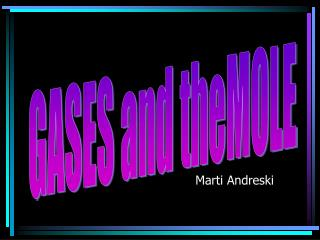DownloadDownload PresentationMarti Andreski

# Marti Andreski

Télécharger la présentation## Marti Andreski

- - - - - - - - - - - - - - - - - - - - - - - - - - - E N D - - - - - - - - - - - - - - - - - - - - - - - - - - -
##### Presentation Transcript

1. GASES and theMOLE Marti Andreski

2. Introduction • the # of gas molec's in a container determines the P at a given T • Avogadro's Principle: "At equal T's and P's, equal V's of gases contain the same # of molecules" • "n" relates to the number of moles of gas at constant T and P, if V1=V2, then n1 = n2

3. Molar Volume @STP • 1 mol of any gas occupies the same V as 1 mol of any other gas • molar volume = the V of 1 mole of a gas at STP = 22.4 L

4. Ideal Gas Equation • P V = n R T • comes from Boyle's Law, Charles's Law, & molar volume • if we substitute in all standard conditions, we can solve for "R"

5. Solving for R • P V = n R T • Rearrange to solve for R • R = PV / nT Memorize These R = (1 atm)(22.4 L) / (1 mol)(273 K) = 0.0821 ____ L. atm/mol K R = (101.3 kPa)(22.4 L) / (1 mol)(273 K) = ___ L. kPa/mol K 8.31 R = (760 torr)(22.4 L) / (1 mol)(273 K) = 62.4 ___ L. torr/mol K

6. Repeat: Memorize all of those values for R! • this crazy unit doesn't really exist as a measurable entity but is used for cancellation purposes

7. Molecular Mass • what are the units for MM? How can you use PV = nRT? • can be found from lab measurements in this way: • MM = m R T • P V • ie: What is the molecular mass of a gas if 150.0 cm3 of it has a mass of 0.922 g at 99.0oC and 107.0 kPa?

8. Molecular Mass Problem • ie: What is the molecular mass of a gas if 150.0 cm3 of it has a mass of 0.922 g at 99.0oC and 107.0 kPa? • MM = m R T • P V

9. And another formula... • Since… • MM = m R T • P V • Then… • MM = DRT • P

10. Gas Stoichiometry at Standard Conditions • main point: at STP, 1 mole of any gas occupies a V of 22.4 L • ie: what V of H2 at STP can be produced from the reaction of 6.54 g of Zn with HCl? • ie: how many g NaCl can be produced by the reaction of 112 cm3 of chlorine at STP with an excess of sodium?

11. Gas Stoichiometry at Non-Standard Conditions • Four types of problems to expect • Each type requires a slightly different approach

12. a) Mass - Volume (Non) • what V of chlorine gas at 24.0oC and 99.2 kPa would be required to react with 2.51 g Ag according to: • 2Ag + Cl2 ---> 2AgCl • i) Stoich “pretending” standard – this would be your answer if conditions were STP • ii) VPT the answer

13. b. Volume (Non) – Mass • What mass of mercury (II) chloride will react with 0.567 dm3 of ammonia at 27oC and 102.7 kPa? • HgCl2 + 2NH3 ---> Hg(NH2)Cl + NH4Cl • i) VPT first • ii) Stoich

14. c. Volume (Non) - Volume (same) • what volume of oxygen at 100oC and 105.5 kPa is required to burn 684 cm3 of methane at the same T and P? • CH4+ 2O2 --> CO2 + 2H20 • i) if T & P do not change, no need to convert; just do Stoich

15. d. Volume (Non) - Volume (Diff) • what volume of oxygen at 26.0oC and 102.5 kPa is required to burn 684 cm3 of methane at 101oC and 107.5 kPa? • CH4+ 2O2 --> CO2 + 2H20 • i) VPT to standard • ii) Stoich • iii) VPT answer to other non-std

16. Summary – Gas Stoichiometry

17. Graham’s Law • "the relative rates at which two gases under identical condit's of T & P will pass thru a small hole vary inversely with the square roots of their molecular masses" • we know: KE1 = KE2 (at same T) • m1v12 = m2v22 • 2 2 • ...and V1 = m2 • V2 m1 • ie: what is the ratio of the speed of H2 to O2 when T for both is equal? • ...so, H2 is moving 3.98 times faster than O2

18. Dalton’s Law of Partial Pressures • the total P in a container is the sum of the partial P's of the gases in the container • see Water Vapor Pressure Table • it is easy to collect gases as they bubble through water. However, if a gas is collected "over water" a correction must be made which accounts for the amount of water vapor present at that T

19. Partial Pressure Problem • ie: a qty of gas is collected over water at 8.00oC in a 353 cm3 vessel. The manometer indicates a P of 84.5 kPa. What V would the dry gas occupy at standard P and 8.00oC? • i) subtract water vapor 84.5 - 1.1 = 83.4 kPa • ii) combined gas law